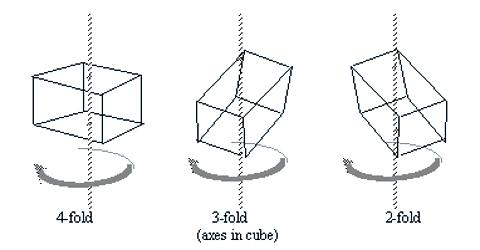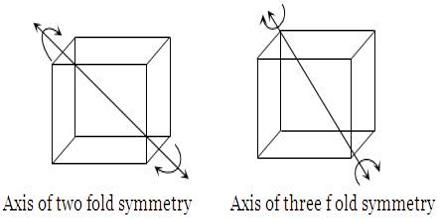# Symmetry in Crystals

Symmetry in Crystals

Symmetry is an important property of crystals. Symmetry in crystals indicates the various ways in which the crystal may be rotated, reflected in a plane or inverted through a point without changing its appearance. Crystals may have;

(i) Plane of symmetry, (ii) axis of symmetry and (iii) centre of symmetry

These are called the elements of symmetry.

(i) Plane of symmetry: When a crystal can be divided by an imaginary plane into two equal parts, each of which is a mirror image of the other, the crystal is said to have a plane of symmetry. A cube has several planes of symmetry, namely those perpendiculars to the faces of the cube and those bisecting the angles of the upper and Lauer faces.

(ii) Axis of symmetry: An axis of symmetry is imaginary line drawn through the crystal about which thee crystal may be rotated so that the crystal shows identical appearance more than once during the rotation through 3600. Such identical appearances may be presented twice, thrice, four tines or six times. Accordingly the crystal is said to possess two-fold, throe-fold, four-fold and six-fold symmetry respectively.

(iii) Centre of symmetry: It is a point at the centre of a crystal. A crystal is said to have a centre of symmetry when any line drawn through this point will intersect the opposite and identical faces, edges or corners of the crystal at equal distances.A crystal may have more than one plane of symmetry and axis of symmetry but never more than one centre of symmetry. All the three types of symmetry are not presented by all crystals. In fact, some crystals do not have any centre of symmetry at all.

The cube has the greatest symmetry. One can examine a cube and find that there are altogether 23 elements of symmetry – thirteen axes of symmetry (three four-fold, four three-fold and six two-fold), nine planes at symmetry and one centre of symmetry. Unless the crystal is perfectly grown it is not always easy to recognize the elements of symmetry but the interfacial angles of a particular crystal system are the same whether the crystal is perfect or imperfect.